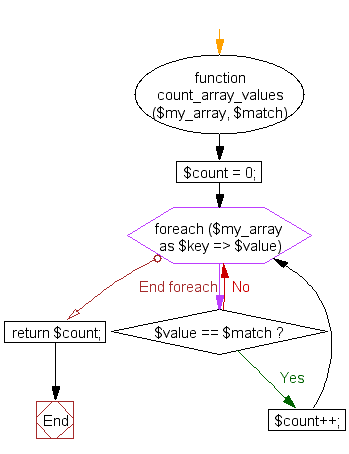﻿ PHP Array Exercise: Count the total number of times a specific value appears in an array - w3resource# PHP Array Exercises : Count the total number of times a specific value appears in an array

## PHP Array: Exercise-37 with Solution

Write a PHP script to count the total number of times a specific value appears in an array.

Sample Solution:

PHP Code:

``````<?php
function count_array_values(\$my_array, \$match)
{
\$count = 0;

foreach (\$my_array as \$key => \$value)
{
if (\$value == \$match)
{
\$count++;
}
}

return \$count;
}

\$colors = array("c1"=>"Red", "c2"=>"Green", "c3"=>"Yellow", "c4"=>"Red");

echo "\n"."Red color appears ".count_array_values(\$colors, "Red"). " time(s)."."\n";
?>
```
```

Sample Output:

```Red color appears 2 time(s).
```

Flowchart:PHP Code Editor:

What is the difficulty level of this exercise?

﻿

## PHP: Tips of the Day

PHP: Extract numbers from a string

```\$str = 'In My Cart : 11 12 items';
preg_match_all('!\d+!', \$str, \$matches);
print_r(\$matches);
```